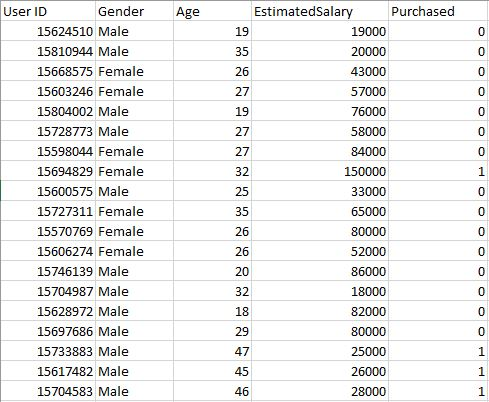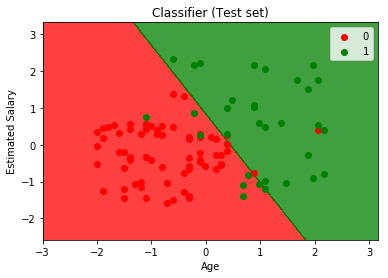# ML | Logistic Regression using Python

• Difficulty Level : Easy
• Last Updated : 09 Jun, 2022

User Database – This dataset contains information about users from a company’s database. It contains information about UserID, Gender, Age, EstimatedSalary, and Purchased. We are using this dataset for predicting whether a user will purchase the company’s newly launched product or not.

Prerequisite: Understanding Logistic Regression

Do refer to the below table from where data is being fetched from the dataset.Let us make the Logistic Regression model, predicting whether a user will purchase the product or not.
Inputting Libraries.

### Import Libraries

```import pandas as pd
import numpy as np
import matplotlib.pyplot as plt```

## Python3

 `dataset ``=` `pd.read_csv(``"User_Data.csv"``)`

Now, to predict whether a user will purchase the product or not, one needs to find out the relationship between Age and Estimated Salary. Here User ID and Gender are not important factors for finding out this.

## Python3

 `# input``x ``=` `dataset.iloc[:, [``2``, ``3``]].values` `# output``y ``=` `dataset.iloc[:, ``4``].values`

### Splitting The Dataset: Train and Test dataset

Splitting the dataset to train and test. 75% of data is used for training the model and 25% of it is used to test the performance of our model.

## Python3

 `from` `sklearn.model_selection ``import` `train_test_split``X_train, X_test, y_train, y_test ``=` `train_test_split(x, y, test_size ``=` `0.25``, random_state ``=` `0``)`

Now, it is very important to perform feature scaling here because Age and Estimated Salary values lie in different ranges. If we don’t scale the features then the Estimated Salary feature will dominate the Age feature when the model finds the nearest neighbor to a data point in the data space.

## Python3

 `from` `sklearn.preprocessing ``import` `StandardScaler``sc_x ``=` `StandardScaler()``xtrain ``=` `sc_x.fit_transform(xtrain)``xtest ``=` `sc_x.transform(xtest)` `print` `(xtrain[``0``:``10``, :])`

Output:

```[[ 0.58164944 -0.88670699]
[-0.60673761  1.46173768]
[-0.01254409 -0.5677824 ]
[-0.60673761  1.89663484]
[ 1.37390747 -1.40858358]
[ 1.47293972  0.99784738]
[ 0.08648817 -0.79972756]
[-0.01254409 -0.24885782]
[-0.21060859 -0.5677824 ]
[-0.21060859 -0.19087153]]```

Here once see that Age and Estimated salary features values are scaled and now there in the -1 to 1. Hence, each feature will contribute equally to decision making i.e. finalizing the hypothesis.

Finally, we are training our Logistic Regression model.

## Python3

 `from` `sklearn.linear_model ``import` `LogisticRegression``classifier ``=` `LogisticRegression(random_state ``=` `0``)``classifier.fit(xtrain, ytrain)`

After training the model, it is time to use it to do predictions on testing data.

## Python3

 `y_pred ``=` `classifier.predict(xtest)`

Let’s test the performance of our model – Confusion Matrix

### Evaluation Metrics

Metrics are used to check the model performance on predicted values and actual values.

## Python3

 `from` `sklearn.metrics ``import` `confusion_matrix``cm ``=` `confusion_matrix(ytest, y_pred)` `print` `(``"Confusion Matrix : \n"``, cm)`

Output:

```Confusion Matrix :
[[65  3]
[ 8 24]]```

Out of 100 :
True Positive + True Negative = 65 + 24
False Positive + False Negative = 3 + 8
Performance measure – Accuracy

Example

## Python3

 `from` `sklearn.metrics ``import` `accuracy_score``print` `(``"Accuracy : "``, accuracy_score(ytest, y_pred))`

Output:

`Accuracy :  0.89`

## Python3

 `from` `matplotlib.colors ``import` `ListedColormap``X_set, y_set ``=` `xtest, ytest``X1, X2 ``=` `np.meshgrid(np.arange(start ``=` `X_set[:, ``0``].``min``() ``-` `1``,``                               ``stop ``=` `X_set[:, ``0``].``max``() ``+` `1``, step ``=` `0.01``),``                     ``np.arange(start ``=` `X_set[:, ``1``].``min``() ``-` `1``,``                               ``stop ``=` `X_set[:, ``1``].``max``() ``+` `1``, step ``=` `0.01``))` `plt.contourf(X1, X2, classifier.predict(``             ``np.array([X1.ravel(), X2.ravel()]).T).reshape(``             ``X1.shape), alpha ``=` `0.75``, cmap ``=` `ListedColormap((``'red'``, ``'green'``)))` `plt.xlim(X1.``min``(), X1.``max``())``plt.ylim(X2.``min``(), X2.``max``())` `for` `i, j ``in` `enumerate``(np.unique(y_set)):``    ``plt.scatter(X_set[y_set ``=``=` `j, ``0``], X_set[y_set ``=``=` `j, ``1``],``                ``c ``=` `ListedColormap((``'red'``, ``'green'``))(i), label ``=` `j)``    ` `plt.title(``'Classifier (Test set)'``)``plt.xlabel(``'Age'``)``plt.ylabel(``'Estimated Salary'``)``plt.legend()``plt.show()`

Output:Analyzing the performance measures – accuracy and confusion matrix and the graph, we can clearly say that our model is performing really well.

My Personal Notes arrow_drop_up